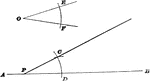### Construction Of Angle On Straight Line

Illustration used to show how to "draw a straight line through any given point on a given straight line…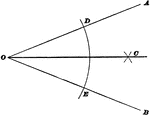### Bisect An Angle

Illustration used to show how to construct a bisector of an angle when the sides intersect within the…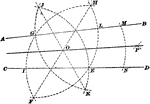### Bisect An Angle

Illustration used to show how to construct a bisector of an angle when the sides no not intersect within…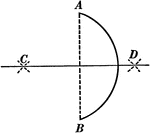### Bisecting an Arc

Illustration used to show how to bisect a given arc.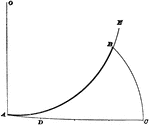### Construction Of Arc

Illustration used to show how to "find an arc of a circle having a known radius, which shall be equal…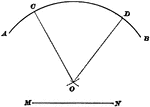### Construction Of Center

Illustration used to show how to find the center when given an arc and its radius.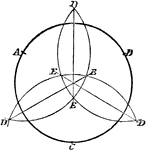### Construction of Circle Given 3 Points

Illustration used to construct a circle when given three points.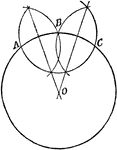### Center of a Circle

Illustration used to find the center of a circle.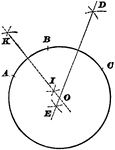### Construction Of Circumference

Illustration used to show how to pass a circumference through any three points not in the same straight…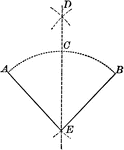### Construction of Bisecting a Given Angle

Illustration of the construction used to bisect a given angle.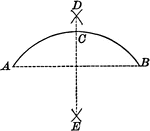### Construction of Bisecting a Given Arc

Illustration of the construction used to bisect a given arc.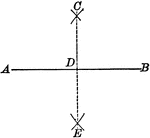### Construction of Bisecting a Given Line

Illustration of the construction used to bisect a given line.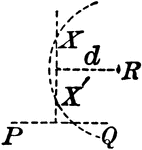### Construction of a Circle When Given Two Points and a Radius

Illustration used to construct a circle when given two points that it passes through and a radius.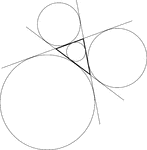### Triangle With Circle Constructions

Illustration of a triangle with its incircle and three excircles constructed.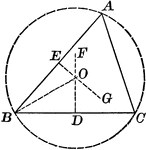### Construction to Circumscribe a Circle About a Triangle

Illustration of the construction used to circumscribe a circle about a given triangle.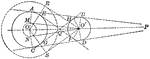### Construction of a Common Tangent When Given Two Circles

Illustration used to construct a common tangent when given two circles.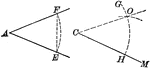### Construction of an Equal Angle Given a Point and a Straight Line

Illustration of the construction used to create an equal angle given a point in a given straight line…### Construction of Escribed Circles With Ex-centres

Illustration of the construction used to escribe circles with centres (centers) called ex-centres of…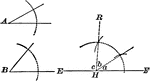### Construction to Find the Third Angle of a Triangle When Given Two Angles

Illustration of the construction used to find the third angle of a triangle when two of the angles are…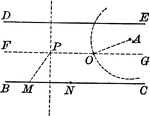### Construction of a Circle Through a Given Point that Cuts Chords of Given Lengths From Parallels

Illustration used to construct a circle that shall pass through a given point and cut chords of a given…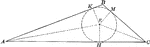### Construction to Inscribe a Circle in a Triangle

Illustration of the construction used to inscribe a circle in a given triangle.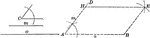### Construction of a Parallelogram When Given Two Sides and Included Angle

Illustration of the construction used to make a parallelogram when given two sides and the included…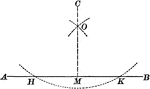### Construction of Perpendicular Upon a Given Line From an External Point

Illustration to let fall a perpendicular upon a given line from a given external point.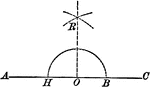### Construction of Perpendicular From a Given Point on a Straight Line

Illustration of the construction of a perpendicular to a line when given a point O on the straight line.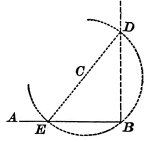### Construction of Perpendicular From a Given Point on a Straight Line

Illustration of the construction of a perpendicular to a line when given a point B on the straight line.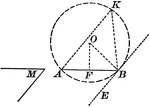### Construction to Describe a Segment of a Circle in Which an Angle Can Be Inscribed

Illustration of the construction used upon a given straight line, to describe a segment of a circle…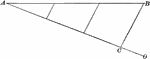### Construction of a Straight Line Divided Into Equal Parts

Illustration of the construction used to divide a straight line into a given number of equal parts.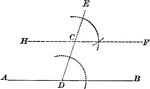### Construction of a Straight Line Parallel to a Given Straight Line

Illustration of the construction used to create straight line parallel to a given straight line through…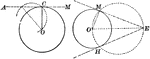### Construction of Tangent Line Through a Given Point to a Given Circle

Illustration of the construction used to draw a tangent to a given circle through a given point.### Construction of a Triangle When Given Two Sides and the Angle Opposite (Ambiguous Case)

Illustration of the construction used to make a triangle when given two sides and the angle opposite…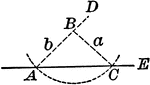### Construction of a Triangle When Given Two Sides and the Angle Opposite (Ambiguous Case)

Illustration of the construction used to make a triangle when given two sides and the angle opposite…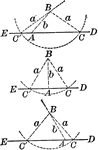### Construction of a Triangle When Given Two Sides and the Angle Opposite (Ambiguous Case)

Illustration of the construction used to make a triangle when given two sides and the angle opposite…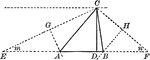### Construction of a Triangle Given Perimeter, Angle, Altitude

Illustration used to construct a triangle , given the perimeter, one angle, and the altitude from the…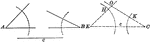### Construction of a Triangle When Given a Side and Two Angles

Illustration of the construction used to make a triangle when given a side and two angles.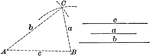### Construction of a Triangle When Given Three Sides

Illustration of the construction used to make a triangle when given three sides.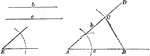### Construction of a Triangle When Given Two Sides and Included Angle

Illustration of the construction used to make a triangle when given two sides and the included angle.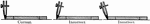### Positioning Pen for Constructions

Illustration showing the correct and incorrect position of a right line pen against a T-square, triangle,…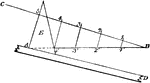### Construction Used to Divide a Line Into Equal Parts

Illustration used to show how to divide a line into any number of equal parts by construction.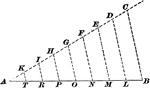### Construction Of Dividing A Line

Illustration used to show how to divide a given straight line into required number of equal parts.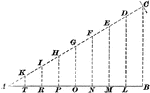### Construction Of Dividing A Line

Illustration used to show how to divide a given straight line into required number of equal parts.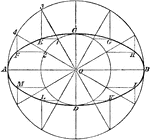### Construction of Ellipse by Describing Circles

Illustration used to draw a an ellipse using string and pins by describing a circles with diameters…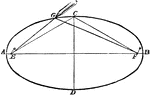### Construction of Ellipse Using String

Illustration used to draw a an ellipse using string and pins.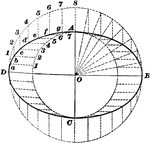### Construction Of Ellipse

Illustration used to show how to draw an ellipse when given the diameters.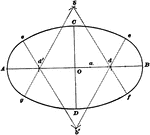### Construction Of Ellipse

Illustration used to show how to draw an ellipse by circular arcs.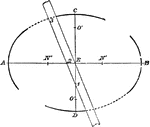### Construction of Ellipse

Illustration used to draw a an ellipse with major axis AB and minor axis CD.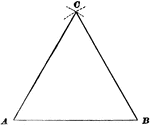### Construction Of Equilateral Triangle

Illustration used to show how to draw an equilateral triangle when given one side.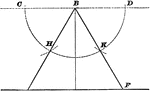### Construction Of Equilateral Triangle

Illustration used to show how to draw an equilateral triangle when given the altitude.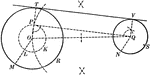### Construction of an External Tangent to 2 Circles

Illustration used to show how "to construct a common external tangent to two given circles."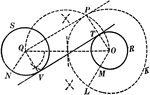### Construction of an 2 External Tangents to 2 Circles

Illustration used to show how to construct two common external tangents to two given circles.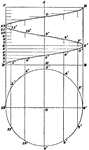### Construction Of Helix

Illustration used to show how to draw a helix when the pitch and the diameter are given.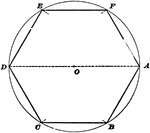### Construction Of Hexagon Inscribed In Circle

Illustration used to show how to inscribe a regular hexagon in a given circle.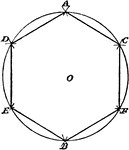### Construction of Regular Hexagon

Illustration used to construct a regular hexagon on a given line.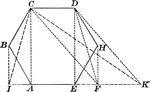### Hexagon Used to Construct Equivalent Triangle

Illustration of a how to construct a triangle equivalent to a given hexagon/polygon.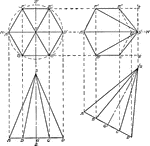### Construction of Hexagonal Pyramid

Construction of a regular hexagonal pyramid.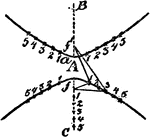### Construction Of A Hyperbola

An illustration showing how to construct a hyperbola by plotting. "Having given the transverse axis…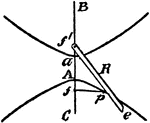### Construction Of A Hyperbola

An illustration showing how to construct a hyperbola by a pencil and a string. "Having given the transverse…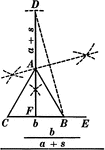### Construction Of An Isosceles Triangle

Illustration of the construction used to create an isosceles triangle, given the bases and the sum of…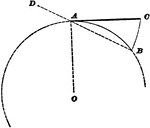### Construction Of Line Equal To Arc

Illustration used to show how to find a straight line of the same length as a given arc of a circle.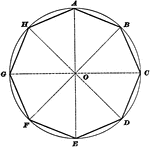### Construction Of Octagon Inscribed In Circle

Illustration used to show how to inscribe a regular octagon in a given circle.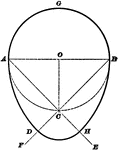### Construction Of Oval

Illustration used to show how to draw an egg-shaped oval when given the diameters.